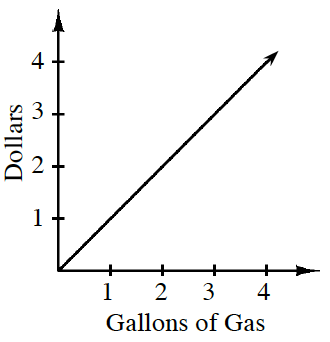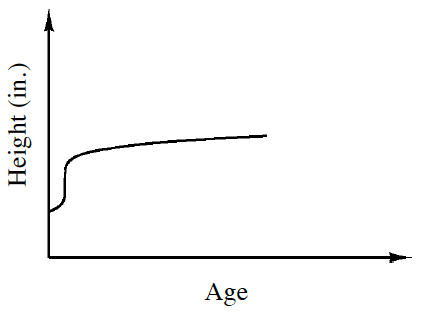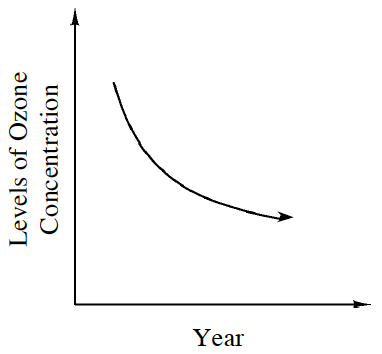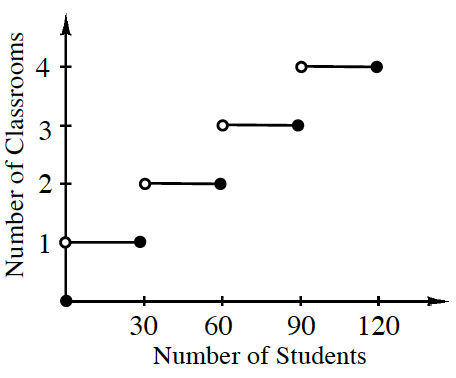### Home > CCA2 > Chapter Ch1 > Lesson 1.1.2 > Problem1-23

1-23.

Examine each graph below. Based on the shape of the graph and the labels of the axes, write a sentence to describe the relationship that each graph represents. Then state which axis represents the independent variable and which one represents the dependent variable.

1.The more gas you buy, the more money you spend.
Gallons are independent and dollars are dependent.

1.People grow a lot in their early years, and then their growth slows. Age is independent and height is dependent.

1.As time goes by, the ozone concentration goes down, although the effect is slowing. The year is independent and the ozone is dependent.

1.As the number of students grows, more classrooms are used and each classroom holds $30$ students.
I: students, D: classrooms.

1. What are all of the possible inputs of the graph in part (d)? What are all of the possible outputs?

Possible inputs: $x$ can be any number between and including $0$ and $120$.
Possible outputs: $y=1,2,3,4$.